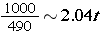# Simple InterestIn this lesson, you will learn to compute simple interest. Interest is the amount of money charged or paid for borrowing or using money. When you deposit money into a savings account, you are paid interest. When you borrow money, you pay interest. Following is the formula used to compute interest.

The interest is the money earned. The principal is the amount of money deposited or borrowed. The rate is the percent that is used and should always be changed to a decimal. The time is calculated in years.

Multiply the principal by the rate by the time to get the interest. Look at a few examples and see how simple it is to use the formula.

### Example 1

Find the interest for the following conditions:

The principal is \$225, the rate is 3%, and the time is 2 years.

First, write the formula.

I = P * r * t    Next, substitute the values given in the conditions.

I = \$225 * .03 * 2    Now, just multiply, using your calculator.

I = \$13.50    The interest is \$13.50.

### Example 2

Find the rate for the following conditions:

The interest is \$300, the Principal is \$1,000, and the time is 5 years.

First, write the formula.

I = P*r*t   Next, substitute the values given in the conditions.

300 = 1000 * 5 * r   Now, multiply the right side to get an equation.

300 = 5000r   As you can see, you only have to divide both sides by 5000 to find r.The rate is 6%.

Now, let us put the conditions into a word problem.

### Example 3

Erica deposits \$7000 in an account that earns 7% simple interest. About how long will it take for her account balance to reach \$8,000?

Write the formula: I = P * r * t

List the information

Principal = 7,000. Rate =0.07   Balance (including interest will be 8,000). This means she will have earned \$1000 interest! Now substitute and solve.

1000 = 7000 * 0.07 * t

1000 = 490tor it will take just a bit over two years for her to get \$8000 in her account with these conditions.

Did you notice that as long as there are three known values, the other value can be found using the formula for simple interest?

Complete this learning activity before moving on to the assignments.

## Simple Interest Practice

Principal Rate Time Interest Total Amt
\$225 5% 3 (1) (1)
\$4250 7% 1.5 (2) (2)
\$397 5% 1 (3) (3)
\$700 6.25% 2 (4) (4)
\$775 8% 1 (5) (5)
\$650 4.5% 2 (6) (6)
\$2975 6% 1 (7) (7)
\$500 9% 3 (8) (8)
\$1422 3% 5 (9) (9)
\$1500 3.85% 6 (10) (10)

(source)